Function Repository Resource:

# AsymptoticCurves

Compute the asymptotic curves of a parameterized surface

Contributed by: Wolfram Staff (original content by Alfred Gray)
 ResourceFunction["AsymptoticCurves"][s,{u,v}] compute the equations for asymptotic curves of a surface s parameterized by variables u and v.

## Details and Options

For a regular surface s, an asymptotic curve is a curve c(t) for which the normal curvature vanishes in the direction c'(t).
The differential equation for the asymptotic curves on a surface is e (u')2 + 2f u'v' +g (v')2=0, where e, f and g are coefficients of the second fundamental form.

## Examples

### Basic Examples (4)

Define a hyperbolic paraboloid:

 In:=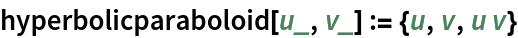The asymptotic curves are just straight lines:

 In:=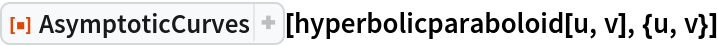Out=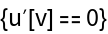The mesh itself represents the asymptotic curves:

 In:=Out=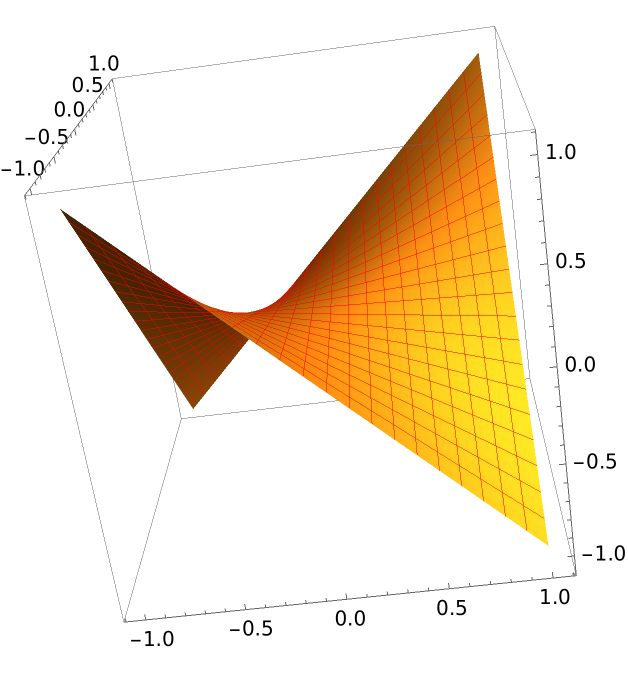Define a funnel:

 In:=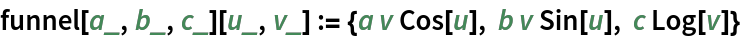Plot it:

 In:=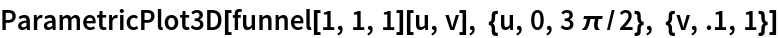Out=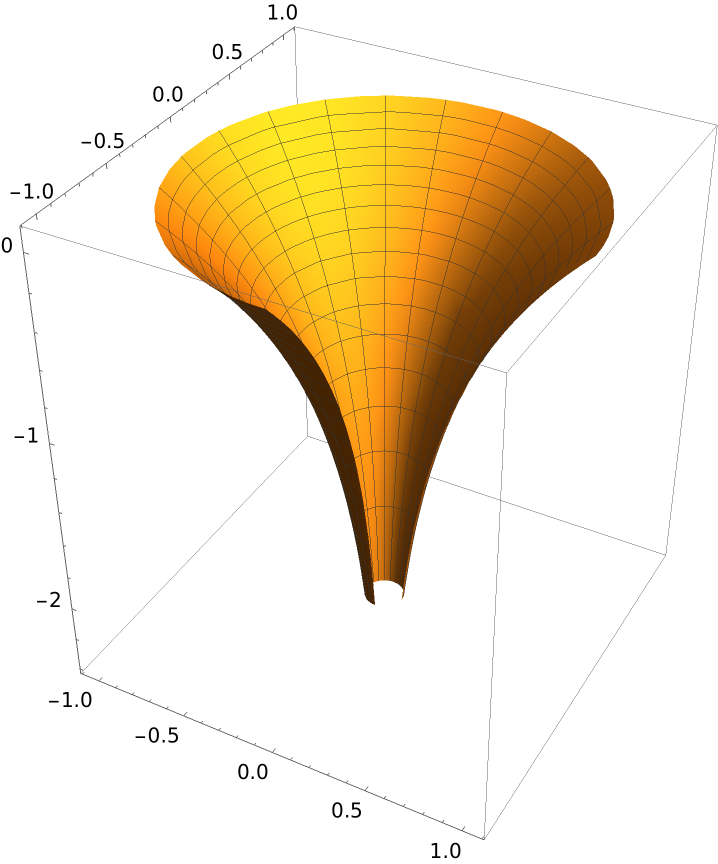Get the equations for asymptotic curves:

 In:=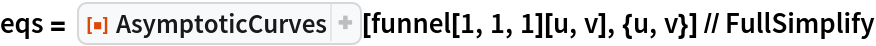Out=Solve the equations:

 In:=Out=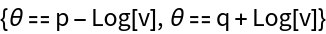Solve for variables:

 In:=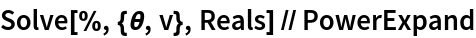Out=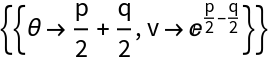Redefine the funnel parameterization in terms of r=ep-q and θ=p+q:

 In:=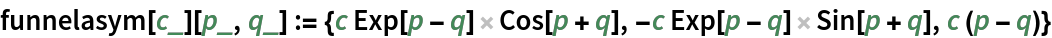Plot the asymptotic curves computed in terms of the new coordinates:

 In:=Out=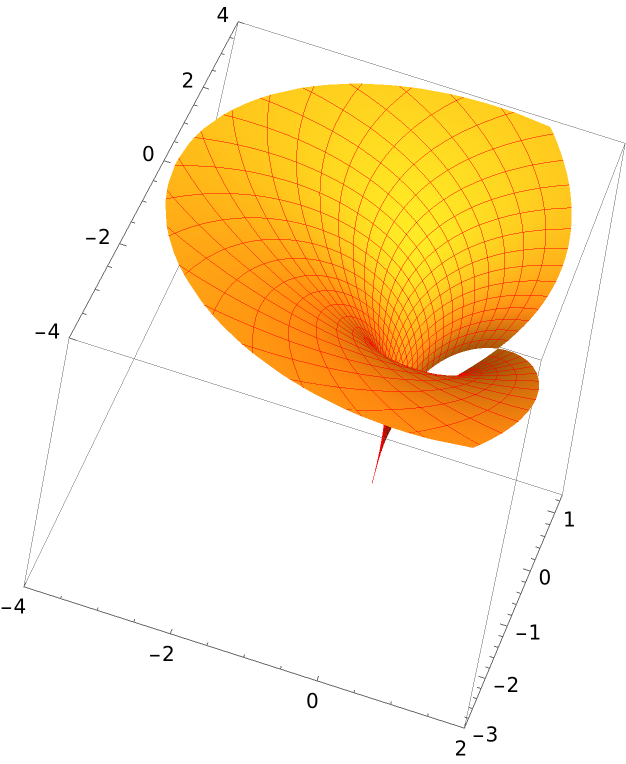Define the exponentially twisted helicoid:

 In:=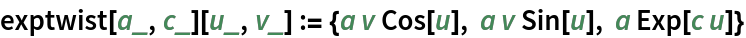Plot the surface:

 In:=Out=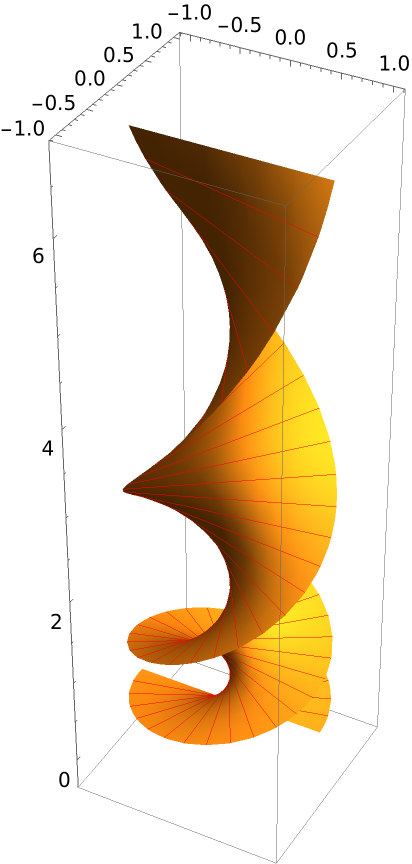The asymptotic curve equations:

 In:=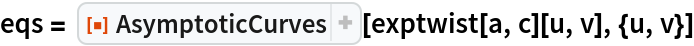Out=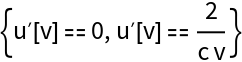The asymptotic curve solutions:

 In:=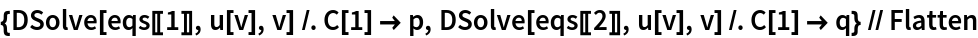Out=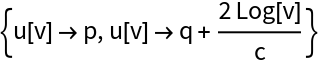Solve for parameters:

 In:=Out=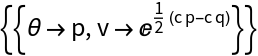Redefine the parameterization in terms of r =ec(p-q) and θ=p:

 In:=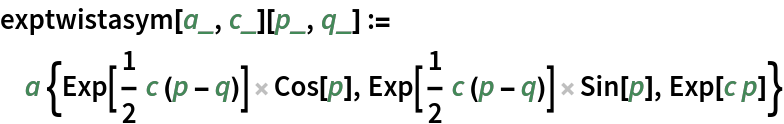Plot the asymptotic curves on the exponentially twisted helicoid:

 In:=Out=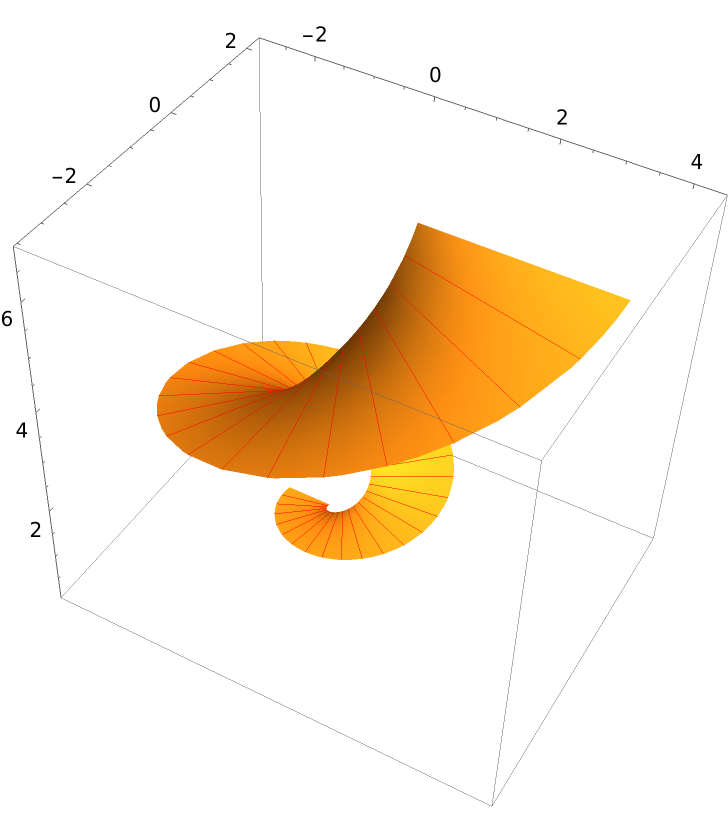Superimpose both the surface and its asymptotic curves:

 In:=Out=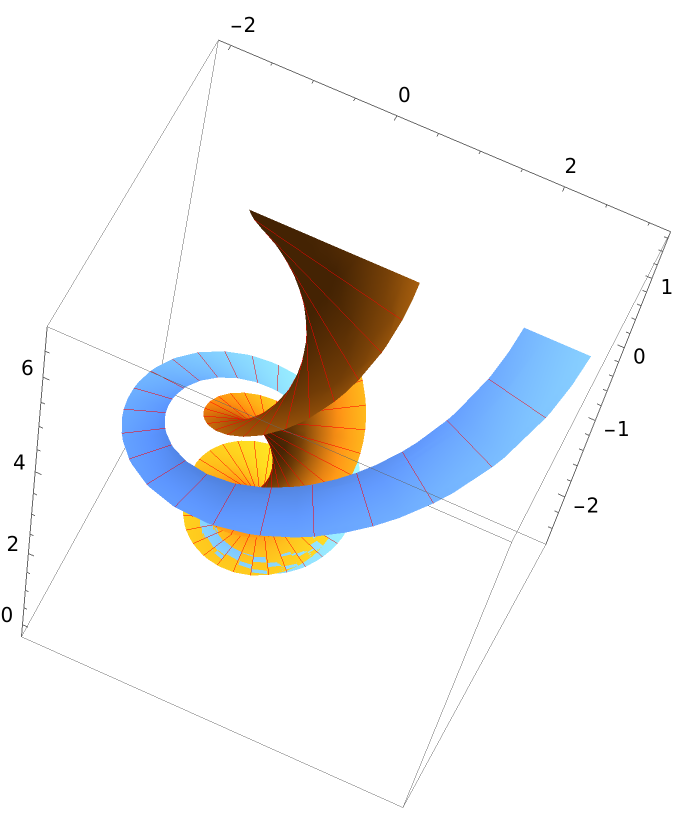Define a wrinkled surface:

 In:=Plot the surface:

 In:=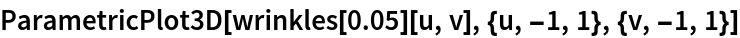Out=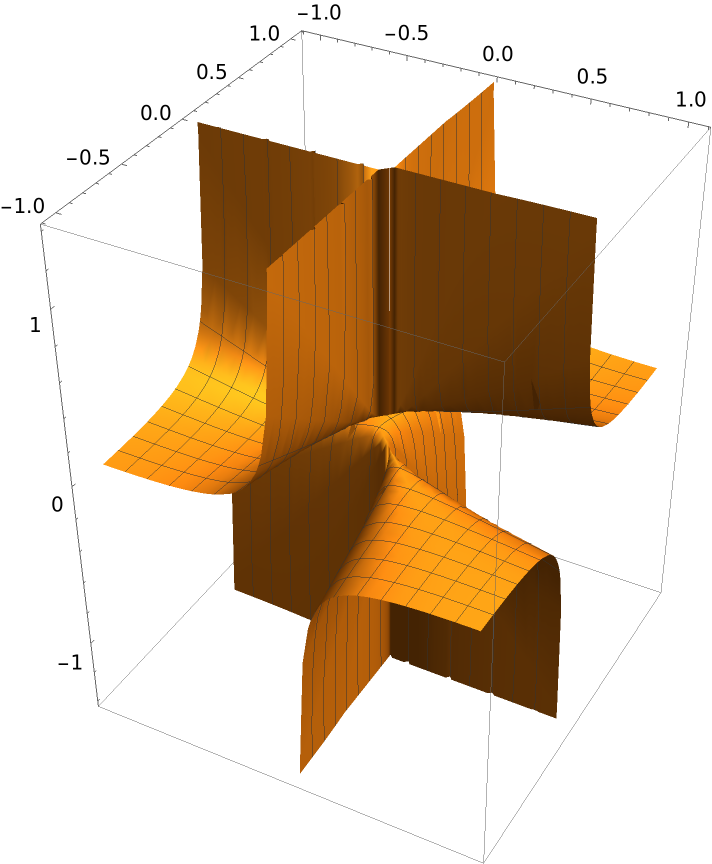Get the equations for asymptotic curves:

 In:=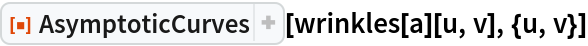Out=Solve the equations:

 In:=Out=Solve for variables:

 In:=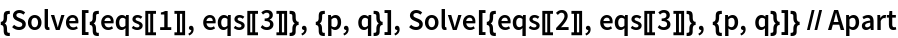Out=A way to visualize the curves using ContourPlot:

 In:=Out=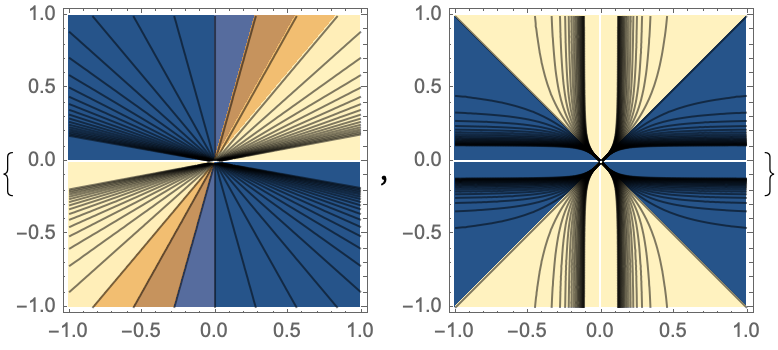### Properties and Relations (7)

Define an elliptic helicoid:

 In:=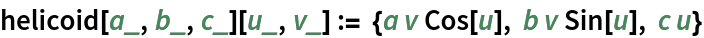The inner and outer edges of the elliptical helicoid are helices:

 In:=Out=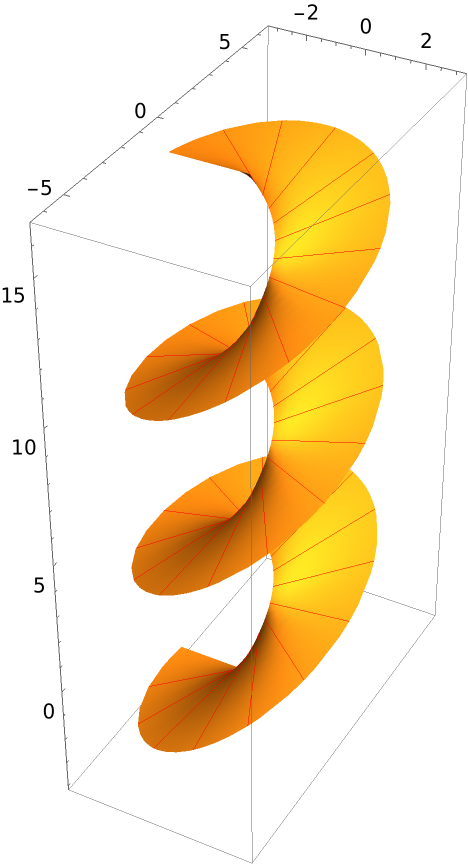Get the asymptotic curve equation:

 In:=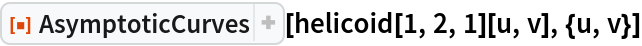Out=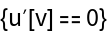For the coefficients of the second fundamental form, only the middle one, f, is nonzero:

 In:=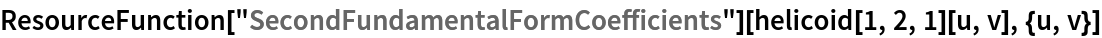Out=Now, verify an instance of the Beltrami-Enneper theorem, starting with the Gaussian curvature:

 In:=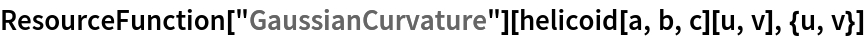Out=Take a helix that is contained in the helicoid:

 In:=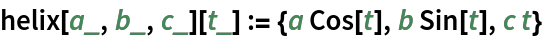Subtract the square of the torsion to give the same expression as shown previously, verifying the identity:

 In:=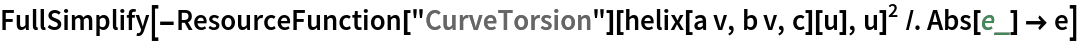Out=Enrique Zeleny

## Version History

• 1.0.0 – 30 July 2020